## Three-Dimensional Navier-Stokes Calculations

The major routine for three-dimensional Navier-Stokes Calculations (compressible and incompressible fluids) is compfluidfem.c. The flow diagram for incompressible and compressible fluids is shown in Figure 173 and 174, respectively. Right now, compfluidfem.c is called once in routine nonlingeo.c. Later on, combined fluid-structure calculations are planned.

The theory behind the fluid calculations is explained in Section 6.9.19. Incompressible fluids (liquids) are calculated using a semi-implicit scheme (the variables compressible and explicit take the value 0), for compressible fluids (gases) an explicit scheme is used (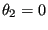, the variables compressible and explicit take the value 1).

Depending on the application different systems of equations have to be solved, corresponding to the transport equations of mass, momentum, total internal energy, turbulent kinetic energy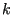and turbulence frequency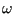. According to the Characteristic Based Split Method (CBS) , a complete increment in time consists of the following steps :

1. First part of the momentum equation: determination of the first time change of the momentum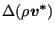2. Conservation of mass: determination of the pressure time change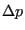for incompressible fluids and the density time change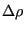for compressible fluids
3. Second part of the momentum equation: determination of the second time change of the momentum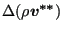4. Conservation of energy: determination of the time change of the total internal energy per unit of volume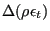5. Turbulence equations: calculation of the time change of the total kinetic energy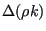and the turbulence frequency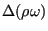The total time change of the momentum is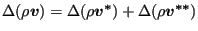. Notice that all variables are written in their conservative form. Indeed, it is not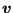which is conserved, but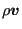and so on.

Each of the above sets leads to a linear equation system to be solved for that increment.

Subsections